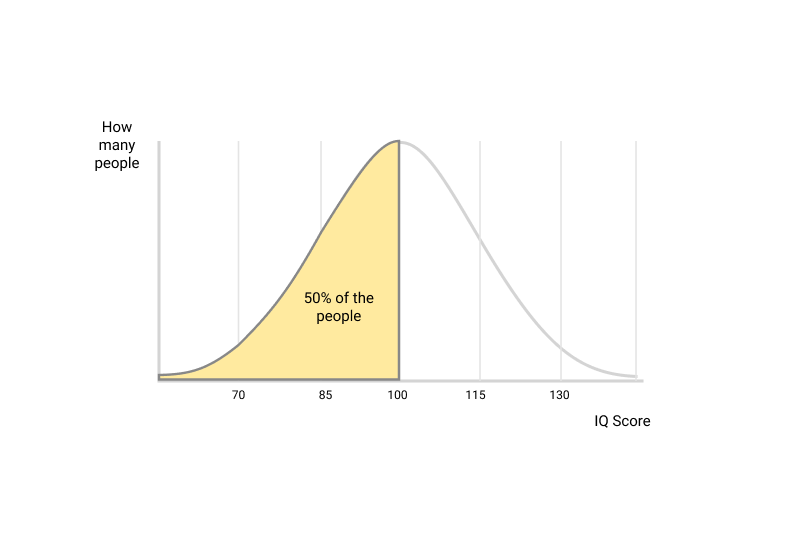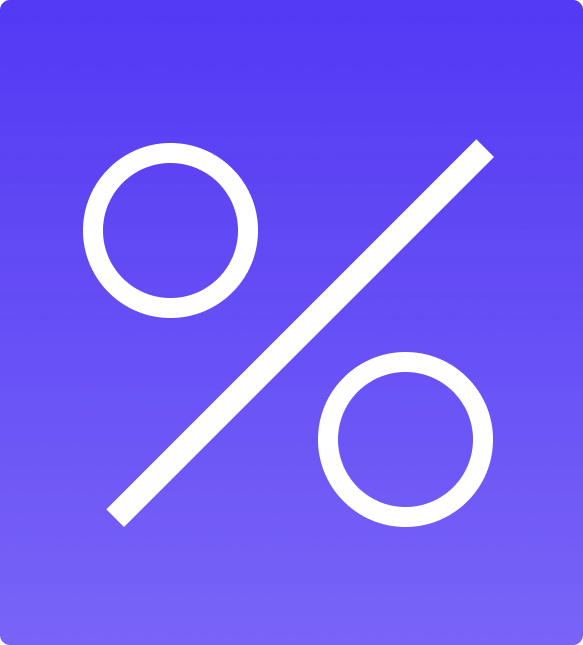# We explain IQ scale, average IQ and ranges

1

## What does IQ mean?

IQ stands for intelligence quotient. It all started with french psychologist Binet. Binet wanted to to calculate whether a child was over or under the average mental development of children of the same age.

To do that, he developed mental tests that were given to children of different years. Then, he calculated the average score for every age. After some hard-work he obtained the IQ averages for each age.

Now when a new child was tested, he would check the performance against children of the same year. If a boy was 14 years old and scored 25 in the test, while the average for his age was scoring 22, Binet concluded that it was more intelligent than the average. He looked into which age had that average, for example let us imagine that a children of 15 years old called Tom scored an average of 25, then he would catalogue the kid as mentally aged 15 years old.

A critical story twist comes from german psychologist W. Stern, who introduced chronological each in order to obtain a good and easily comparable number. IQ as a concept was born. To calculate it, he would divide scored age (which age had for average the score achieved by the kid) by real age. In Tom's example, 15 by 14, which is 1,07.

Since working with decimals was inconvenient, he introduced a multiplication by 100, to avoid them. So Tom obtained 107 of IQ for someone of his age.

However, when psychologists started using the IQ test for adults they soon understood that age was not relevant so they needed another way of comparing intelligence between adults. To do that, they simply compared to a sample of other adults, they painted them in a bell curve and used the IQ Scale, let's us understand it in the next section.
2

## What is the IQ scale?

Summary: usually the IQ scale has mean 100 and standard deviation 15. Let's see why.

This was painted in the famous Bell Score. In one axis (the x axis) the score level and on the other (the y axis) the amount of people that participated in the test and received that score.Different tests had different averages and standard deviations (by the way standard deviation is what is the normal difference between any score taken randomly and the average). For example, let us imagine a test with 50 items and a test with 150 items. As you can guess the average score in the first test could be 35 but in the second test maybe it is 100. On the other hand, what is the usual difference of any random score with the average? Maybe in the first test is 1 point but in the second test is 3 points. How can we compare them? We can't unless we transform the scores.

When painted all scores from all tests they looked the same, most people had average intelligence, fewer on extremes. To find common ground they "decided to use a scale, the average would always be 100 and the standard deviation 15". This way scores are always comparable.

To get to the scale it's a pretty easy two-step process. First, you get the score from the test, deduct the test's average and divide it by the standard deviation. That is a normalized score. You could already compare between tests but we want to rescale to use the typical scale.

Example: imagine a score of 39 in a test with average 35 and standard deviation of 2 -> (39-35) / 1 = 2. The normalized score is "2".

The second step is to simply rescale back to IQ typical scale with average 100 and standard deviation of 15 -> ( 2 * 15 ) + 100 = 110. Perfect, now that it is clear let us recheck average IQ or jump to percentile.
3

## Average IQ?

100
Average IQ
Since IQ was first used as a quotient (scored mental age by real age) the average IQ was always 100, because if you scored as the average and you divide it by the average score, you obtain 1, which as we said was multiplied by 100 to avoid decimals. As we know from the IQ scale section, now we calculate with the Bell curve and the score in the middle is always 100. Could it be any other cypher? Yes, absolutely. If scientists decided to use a different scale they could say "ok, let's use an an average of 50 or of 200". But it wouldn't change anything.

Since IQ scores always mean what they mean because they are a comparison with other scores and people. If the average was 50 then 90 would be genius. That is only a matter of which average and standard deviation we want to use in the scale. More important is IQ percentile, let's learn it.
4

## The IQ PercentileIn truth, the most important and easiest to understand number in IQ calculations is the percentile. The percentile is the percentage of the population that has lower intelligence compared to you (or the person who did the test). Or in other words, which percentage of the population you beat in intelligence.

Since the average IQ is 100, and by definition an average lies in the middle, anyone with 100 IQ sits in the 50th percentile, or in other words, beats 50% of the adult population -or population of its age if children-.
5

## IQ Ranges

The IQ Ranges are simply the score limits -maximum and minimum- to belong to a category of intelligence, be it genius, intermediate or low. Let’s see below the most typical IQ categories:
IQ Score
Minimum IQ
Percentile
Genius
145
99.9%
Very high
130
98%
High
120
90%
Middle-high
108
70%
Middle-low
91
40%
Low
86
20%
VL‍
70
2%